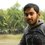# A Problem need to solve.....

I need to know how to solve this problem. Can anyone help me?

In trapezium ABCD, ADIIBC, AD<BC,unparallel sides are equal.A circle with centre O is inscribed in the trapezium. OAD is equilateral. Find the radius of the circle if the area of the trapezium is $\frac{800} {\sqrt{3}}$Note by Partho Kunda
6 years, 7 months ago

This discussion board is a place to discuss our Daily Challenges and the math and science related to those challenges. Explanations are more than just a solution — they should explain the steps and thinking strategies that you used to obtain the solution. Comments should further the discussion of math and science.

When posting on Brilliant:

• Use the emojis to react to an explanation, whether you're congratulating a job well done , or just really confused .
• Ask specific questions about the challenge or the steps in somebody's explanation. Well-posed questions can add a lot to the discussion, but posting "I don't understand!" doesn't help anyone.
• Try to contribute something new to the discussion, whether it is an extension, generalization or other idea related to the challenge.

MarkdownAppears as
*italics* or _italics_ italics
**bold** or __bold__ bold
- bulleted- list
• bulleted
• list
1. numbered2. list
1. numbered
2. list
Note: you must add a full line of space before and after lists for them to show up correctly
paragraph 1paragraph 2

paragraph 1

paragraph 2

[example link](https://brilliant.org)example link
> This is a quote
This is a quote
    # I indented these lines
# 4 spaces, and now they show
# up as a code block.

print "hello world"
# I indented these lines
# 4 spaces, and now they show
# up as a code block.

print "hello world"
MathAppears as
Remember to wrap math in $$ ... $$ or $ ... $ to ensure proper formatting.
2 \times 3 $2 \times 3$
2^{34} $2^{34}$
a_{i-1} $a_{i-1}$
\frac{2}{3} $\frac{2}{3}$
\sqrt{2} $\sqrt{2}$
\sum_{i=1}^3 $\sum_{i=1}^3$
\sin \theta $\sin \theta$
\boxed{123} $\boxed{123}$

Sort by:

$\dfrac{10\sqrt{6}}{3}$?

- 6 years, 7 months ago

I really don't know. Do u know how to solve it?

- 6 years, 7 months ago

This was a 2013 secodary level olympiad problem in Khulna

- 6 years, 6 months ago

You're right.....Good memory of course.......

- 6 years, 6 months ago

You qualified that time?

- 6 years, 6 months ago

Yeah! 1st Runners up.....I qualified in the regional for 4 times.....

- 6 years, 6 months ago

This will be my 1st time

- 6 years, 6 months ago

$\frac { 20 }{ \sqrt { 3 } }$ should be the answer. (by the way, I wrote up a solution and God knows where I made the mistake in LATEXing. Everything started looking like Hebrew :P )

- 6 years, 7 months ago

I really don't know. Do u know how to solve it?

- 6 years, 7 months ago

drop perpendiculars from A & D to BC and call the feet M and N respectively. Let r be the radius and AD = a Say, the circle touches AB . BC , CD , DA at P, Q, R, S respectively, Here AM = 2r and AS = MQ = a/2

Now, OS is the height of equilateral triangle AOD , so OS =r = (root3)a/2 and, AB = AP + PB = AS + BQ = AS + BM +MQ = a + BM [ as AS = MQ = a/2 ] now use Pythagoras in ABM and you'll get a relation between BM and r

so, area of ABCD = ABM + DNC + AMND = 2ABM + AMND [since AMB and DNC are congruent ] Solve the equation and you'll get the answer

(Hope you'll understand..... I messed up again trying to LATEX :P )

- 6 years, 7 months ago

why AP+PB=AS+BQ

- 6 years, 7 months ago

If two tangents are drawn to a circle from an external point, the distances from that point to the points of contact are equal.

- 6 years, 6 months ago

oh! I forgot it.....shit.......and thanks......

- 6 years, 6 months ago